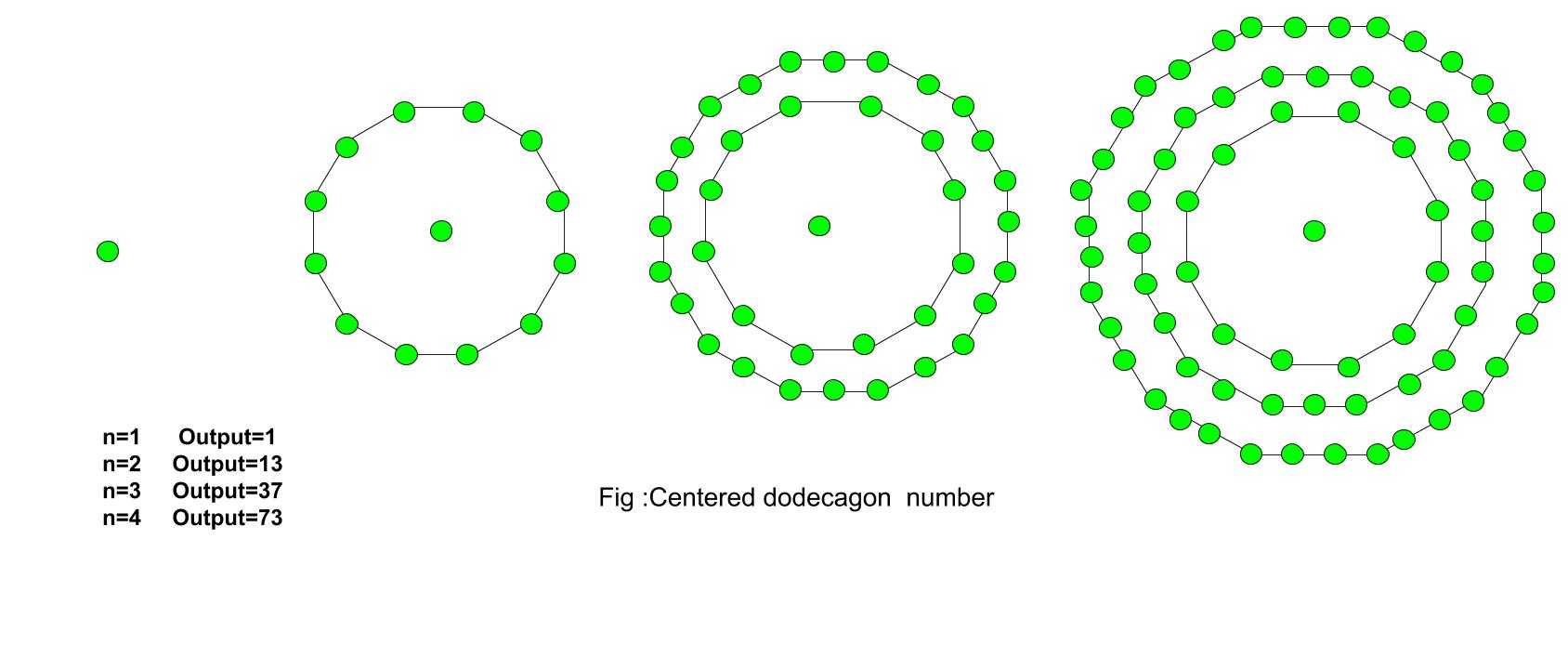# Centered Dodecagonal Number

Given a number n, find the nth Centered Dodecagonal Number.
The Centered Dodecagonal Number represents a dot in the center and other dots surrounding it in successive dodecagonal(12 sided polygon) layers.

Examples :

```Input :  3
Output : 37

Input : 7
Output :253
```The first few centered dodecagonal numbers are:
1, 13, 37, 73, 121, 181, 253, 337, 433, 541, 661…………………..

The formula for the nth Centered dodecagonal number:

``````

## C++

 `// C++ Program to find ` `// nth centered ` `// Dodecagonal number ` `#include ` `using` `namespace` `std; ` ` `  `// Function to calculate Centered ` `// Dodecagonal number ` `int` `centeredDodecagonal(``long` `int` `n) ` `{ ` `    ``// Formula to calculate nth ` `    ``// centered Dodecagonal number ` `    ``return` `6 * n * (n - 1) + 1; ` `} ` ` `  `// Driver Code ` `int` `main() ` `{ ` `    ``long` `int` `n = 2; ` `    ``cout << centeredDodecagonal(n); ` `    ``cout << endl; ` `    ``n = 9; ` `    ``cout << centeredDodecagonal(n); ` ` `  `    ``return` `0; ` `} `

## Java

 `// Java Program to find nth  ` `// centered dodecagonal number ` `import` `java.io.*; ` ` `  `class` `GFG{ ` `     `  `// Function to calculate ` `// centered dodecagonal number ` `static` `long` `centeredDodecagonal(``long` `n) ` `{ ` `     `  `    ``// Formula to calculate nth ` `    ``// centered dodecagonal number ` `    ``return` `6` `* n * (n - ``1``) + ``1``; ` `} ` ` `  `// Driver Code ` `public` `static` `void` `main(String[] args) ` `{ ` `    ``long` `n = ``2``; ` `    ``System.out.println(centeredDodecagonal(n)); ` ` `  `    ``n = ``9``; ` `    ``System.out.println(centeredDodecagonal(n)); ` `} ` `} ` ` `  `// This code is contributed by anuj_67 `

## Python3

 `# Python3 program to find nth  ` `# centered dodecagonal number  ` ` `  `# Function to calculate  ` `# centered dodecagonal number  ` `def` `centeredDodecagonal(n) : ` `     `  `    ``# Formula to calculate nth  ` `    ``# centered dodecagonal number  ` `    ``return` `6` `*` `n ``*` `(n ``-` `1``) ``+` `1``;  ` ` `  `# Driver code ` `n ``=` `2` `print``(centeredDodecagonal(n)); ` ` `  `n ``=` `9` `print``(centeredDodecagonal(n));  ` ` `  `# This code is contributed by grand_master `

## C#

 `// C# Program to find nth  ` `// centered dodecagonal number ` `using` `System; ` `class` `GFG{ ` ` `  `// Function to calculate ` `// centered dodecagonal number ` `static` `long` `centeredDodecagonal(``long` `n) ` `{ ` `     `  `    ``// Formula to calculate nth ` `    ``// centered dodecagonal number ` `    ``return` `6 * n * (n - 1) + 1; ` `} ` ` `  `// Driver Code ` `public` `static` `void` `Main(String[] args)  ` `{  ` `    ``long` `n = 2; ` `    ``Console.WriteLine(centeredDodecagonal(n)); ` `     `  `    ``n = 9; ` `    ``Console.WriteLine(centeredDodecagonal(n)); ` `} ` `} ` ` `  `// This code is contributed by shivanisinghss2110 `

Output :

```13
433
```

References
http://oeis.org/A003154

Attention reader! Don’t stop learning now. Get hold of all the important DSA concepts with the DSA Self Paced Course at a student-friendly price and become industry ready.

My Personal Notes arrow_drop_upCheck out this Author's contributed articles.

If you like GeeksforGeeks and would like to contribute, you can also write an article using contribute.geeksforgeeks.org or mail your article to contribute@geeksforgeeks.org. See your article appearing on the GeeksforGeeks main page and help other Geeks.

Please Improve this article if you find anything incorrect by clicking on the "Improve Article" button below.